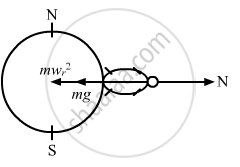Share

# A Person Stands on a Spring Balance at the Equator. If the Speed of Earth'S Rotation is Increased by Such an Amount that the Balance Reading is Half the True Weight, What Will Be the Length - Physics

ConceptCircular Motion

#### Question

A person stands on a spring balance at the equator. If the speed of earth's rotation is increased by such an amount that the balance reading is half the true weight, what will be the length of the day in this case?

#### Solution

When the balance reading is half, we have :

$\text { True weight }= \frac{\text{mg - m }\omega^2 r}{\text{mg}} = \frac{1}{2}$$\Rightarrow \omega^2 r = \frac{g}{2}$

$\Rightarrow \omega = \sqrt{\frac{g}{2r}}$

$= \sqrt{\frac{10}{2 \times 6400 \times {10}^3}} \text{rad/s}$

$\therefore \text { Duration of the day }= 2\pi \times \sqrt{\frac{2 \times 6400 \times {10}^3}{9 . 8}}$s

$= 2\pi\sqrt{\frac{6 . 4 \times {10}^7}{49}}$s

$= \frac{2\pi \times 8000}{7 \times 3600} \text{ h = 2 h}$

Is there an error in this question or solution?

#### APPEARS IN

Solution A Person Stands on a Spring Balance at the Equator. If the Speed of Earth'S Rotation is Increased by Such an Amount that the Balance Reading is Half the True Weight, What Will Be the Length Concept: Circular Motion.
S